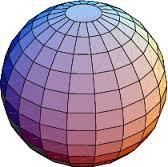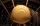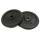# Iron sphere

Iron sphere has weight 100 kg and density ρ = 7600 kg/m3. Calculate the volume, surface, and diameter of the sphere.

V =  13.1579 dm3
D =  2.9291 dm
S =  26.9531 dm2

### Step-by-step explanation:Did you find an error or inaccuracy? Feel free to write us. Thank you!Tips to related online calculators
Do you want to convert length units?
Do you know the volume and unit volume, and want to convert volume units?
Tip: Our Density units converter will help you with the conversion of density units.
Do you want to convert mass units?

#### You need to know the following knowledge to solve this word math problem:

We encourage you to watch this tutorial video on this math problem:

## Related math problems and questions:

• SphereThe surface of the sphere is 12100 cm2, and the weight is 136 kg. What is its density?
• Iron ballThe iron ball weighs 100 kilograms. Calculate the volume, radius, and surface if the iron's density is h = 7.6g/cm3.
• Wall thicknessThe hollow metal ball has an outside diameter of 40 cm. Determine the wall thickness if the weight is 25 kg and the metal density is 8.45 g/cm3.
• Hollow sphereThe steel hollow sphere floats on the water plunged into half its volume. Determine the outer radius of the sphere and wall thickness, if you know that the weight of the sphere is 0.5 kg and the density of steel is 7850 kg/m3
• Iron castWhat is the weight of cast iron with a volume of 3575 cubic centimeters? The density of the cast iron is 7600 kg/m3
• Cone from cubeFrom a wooden block, 20 cm high was the turned largest possible cone. Calculate its weight if you know that the density of wood was 850 kg/m3
• 3d printer3D printing ABS filament with diameter 1.75 mm has density 1.04 g/cm3. Find the length of m = 5 kg spool filament. (how to calculate length)
• SeawaterSeawater has a density of 1025 kg/m3, ice 920 kg/m3. 8 liters of seawater froze and created a cube. Calculate the size of the cube edge.
• Area of a cubeCalculate the surface area of a cube if its volume is equal to 729 cubic meters.
• Cuboid 5Calculate the mass of the cuboid with dimensions of 12 cm; 0.8 dm and 100 mm made from spruce wood (density = 550 kg/m3).
• Cu wireCopper wire has a length l = 820 m and diameter d = 10 mm. Calculate the weight if density of copper is ρ = 8500 kg/m3. Result round to one decimal place.
• Cube surface and volumeThe surface of the cube is 500 cm2, how much cm3 will be its volume?
• Iron densityCalculate the weight of a 2 m long rail pipe with an internal diameter of 10 cm and a wall thickness of 3 mm. The iron density is p = 7.8 g/cm3.
• The cubeThe cube has a surface area of 216 dm2. Calculate: a) the content of one wall, b) edge length, c) cube volume.
• Plastic pipeCalculate the plastic pipe's weight with diameter d = 70 mm and length 380 cm if the wall thickness is 4 mm and the density of plastic is 1367 kg/m3.
• Steel tubeThe steel tube has an inner diameter of 4 cm and an outer diameter of 4.8 cm. The density of the steel is 7800 kg/m3. Calculate its length if it weighs 15 kg.
• Sphere A2VThe surface of the sphere is 241 mm2. What is its volume?